# 手把手带你用Numpy实现CNN <零>

Numpy是一个非常好用的python科学计算的库，CNN是现在视觉领域深度学习的基础之一。虽然好的框架很多，不过自己用Numpy实现一个可以使用的CNN的模型有利于初学者加深对CNN的理解。

## 内容介绍：

• Numpy实现基本的CNN网络组件:conv, pooling, relu, fullyconnect, softmax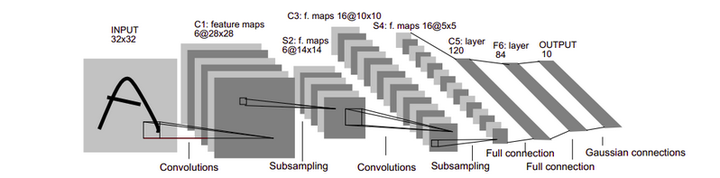LeNet网络结构图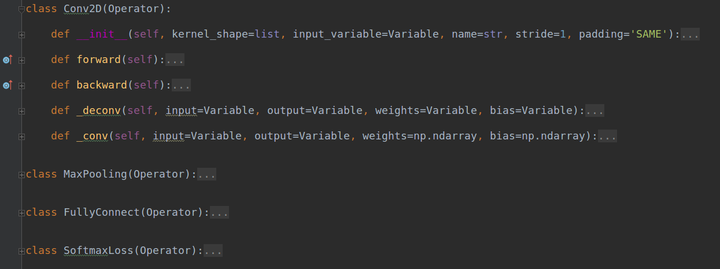基本组件列表
• 从实现的基本组件搭建可以训练的网络，完成mnist的训练与测试，画出一个下图的训练曲线
• 从贯序连接的层模型到计算图模型的引入，完成自动求值，求导等功能
• 常见的初始化方法，激活函数，优化方法，Loss函数的实现与比较，例如relu系与sigmoid系的比较，sgd,momentum,Adam的比较等等
• 常见的trick的实现，例如dropout, batchnorm, residual

## 开始之前：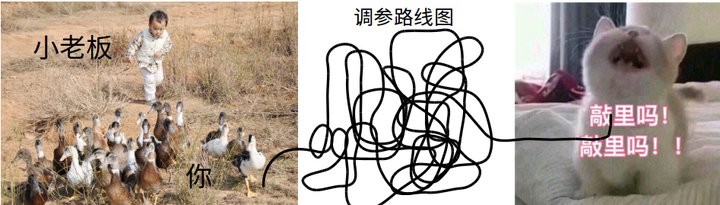从赶鸭子上架到踹门自学

1. 如果你会一点Python(~不会也没关系,贼简单)
2. 如果你也想入门深度学习（～大概知道lenet+bp就可以了）

## 卷积层（Convolutions）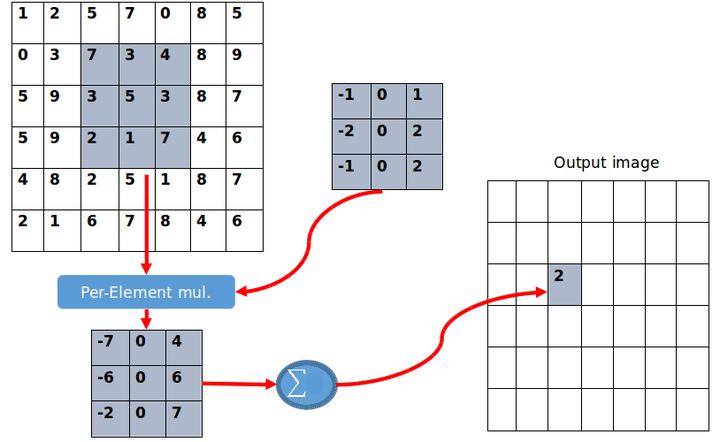卷积计算图解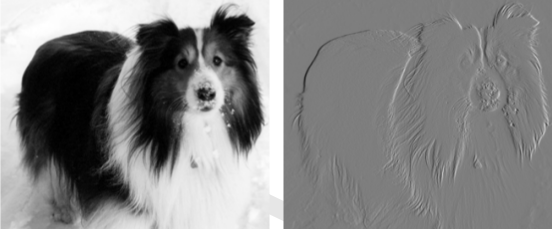卷积计算效果图

1. 一次操作（一层）中使用多个卷积核得到该尺度下的多张特征映射
2. 多层（次）提取不同尺度下的不同特征信息## Show me your code

PS:文章看到的版本是不基于graph模型的，Conv2D直接继承自Object,所有的数据和操作都是裸露的，单纯为了实现功能。github上面这一部分代码已经不用了，放在layers文件夹下。

1. 输入数据的shape = [N,W,H,C] N=Batchsize/W=width/H=height/C=channels
2. 卷积核的尺寸ksize ,个数output_channels, kernel shape [output_channels,k,k,C]
3. 卷积的步长，基本默认为1.
4. 卷积的方法，VALID or SAME，即是否通过padding保持输出图像与输入图像的大小不变

class Conv2D(object):    def __init__(self, shape, output_channels, ksize=3, stride=1, method='VALID'):        self.input_shape = shape        self.output_channels = output_channels        self.input_channels = shape[-1]        self.batchsize = shape        self.stride = stride        self.ksize = ksize        self.method = method        weights_scale = math.sqrt(ksize*ksize*self.input_channels/2)        self.weights = np.random.standard_normal(    (ksize, ksize, self.input_channels, self.output_channels)) / weights_scale        self.bias = np.random.standard_normal(self.output_channels) / weights_scale


conv1 = Conv2D([batch_size, 28, 28, 1], 12, 5, 1)


if method == 'VALID':    self.eta = np.zeros((shape, (shape - ksize ) / self.stride + 1, (shape - ksize ) / self.stride + 1,     self.output_channels))
if method == 'SAME':    self.eta = np.zeros((shape, shape/self.stride, shape/self.stride,self.output_channels))
self.w_gradient = np.zeros(self.weights.shape) self.b_gradient = np.zeros(self.bias.shape) self.output_shape = self.eta.shape


面试官：     ”...天南...海北...“ 面试官：     ”你知道卷积是怎么算的嘛？“（原话） 懵逼的我：   ”还能怎么算～？～不就是点乘么？“ 面试官：     ～懵逼～ 我：         ～懵逼～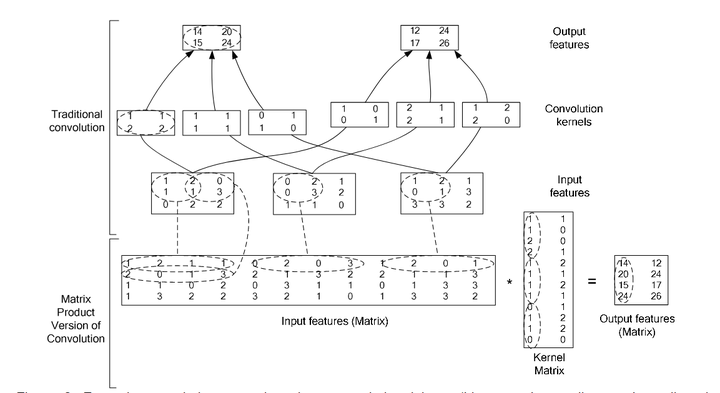图解im2col

def forward(self, x):    col_weights = self.weights.reshape([-1, self.output_channels])    if self.method == 'SAME':        x = np.pad(x, ((0, 0), (self.ksize / 2, self.ksize / 2), (self.ksize / 2, self.ksize / 2), (0, 0)),                         'constant', constant_values=0)    self.col_image = []    conv_out = np.zeros(self.eta.shape)    for i in range(self.batchsize):        img_i = x[i][np.newaxis, :]        self.col_image_i = im2col(img_i, self.ksize, self.stride)        conv_out[i] = np.reshape(np.dot(self.col_image_i, col_weights) + self.bias, self.eta.shape)        self.col_image.append(self.col_image_i)    self.col_image = np.array(self.col_image)    return conv_out

1. 首先我们将卷积层的参数weights通过ndarray自带的reshape方法reshape到上图中Kernal Matrix的形状。
2. 根据self.method，选择是否对输入的数据进行padding,这里我们调用方法，对我们的输入数据四维ndarray的第二维和第三维分别padding上与卷积核大小相匹配的0元素
3. 声明一个list用于存储转换为column的image,在backward中我们还会用到
4. 对于batch中的每一个数据，分别调用im2col方法，将该数据转化为上图中的Input features(Matrix), 然后调用完成矩阵乘法得到Output features(Matrix), reshape输出的shape,填充到输出数据中。

im2col的代码如下：

def im2col(image, ksize, stride):    # image is a 4d tensor([batchsize, width ,height, channel])    image_col = []    for i in range(0, image.shape - ksize + 1, stride):        for j in range(0, image.shape - ksize + 1, stride):            col = image[:, i:i + ksize, j:j + ksize, :].reshape([-1])            image_col.append(col)    image_col = np.array(image_col)    return image_colNumpy是一个非常好用的python科学计算的库，CNN是现在视觉领域深度学习的基础之一。虽然到2019年，好的框架已经有很多，不过自己用Numpy实现一个可以使用的CNN的模型有利于初学者加深对CNN的理解。本文旨在通过这样一个专栏（文章) 介绍如何用Numpy从零实现一个可以训练的CNN简易网络，同时对深度学习(CNN)的相关基础知识进行一些复习。

LeNet技术

LeNet 诞生于 1994 年，是最早的卷积神经网络之一，并且推动了深度学习领域的发展。自从 1988 年开始，在许多次成功的迭代后，这项由 Yann LeCun 完成的开拓性成果被命名为 LeNet5。LeNet5 的架构基于这样的观点：（尤其是）图像的特征分布在整张图像上，以及带有可学习参数的卷积是一种用少量参数在多个位置上提取相似特征的有效方式。在那时候，没有 GPU 帮助训练，甚至 CPU 的速度也很慢。因此，能够保存参数以及计算过程是一个关键进展。这和将每个像素用作一个大型多层神经网络的单独输入相反。LeNet5 阐述了那些像素不应该被使用在第一层，因为图像具有很强的空间相关性，而使用图像中独立的像素作为不同的输入特征则利用不到这些相关性。

zhihu.com